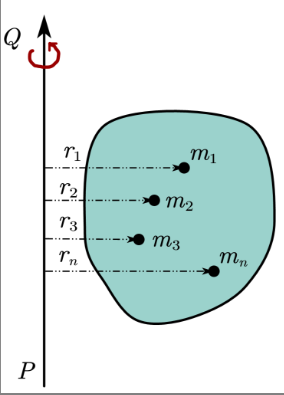## Radius of Gyration formula and explanation

Radius of gyration or gyradius is an important concept and find its applications in structural engineering, mechanics. It even find its applications in polymer physics where the radius of gyration is used to describe the dimensions of a polymer chain(wikipedia).
Radius of gyration also have mathematical definition. Mathematically, radius of gyration $k$ is the root mean square distance of the particles of the body either from its center of mass or from the axis of rotation, depending on the relevant application.

Radius of gyration generally shows up in two places:

1. Strength of materials: Here two dimensional radius of gyration is used and is defined as area property. Here in this case radius of gyration is given by the relation $k=\sqrt{\frac{I}{A}}$ In this case the moment of inertia $I$ is the area moment of inertia. Area moment of inertia is a property of a two-dimensional plane shape which characterizes its deflection under loading.
Here in mechanics we will not concern ourselves with $k$ calculated using area moment of inertia. This variation finds its applications in engineering (Area Moment of Inertia).
2. Mechanics: Here radius of gyration about an axis of rotation is calculated using mass moment of inertia and its formula is given by relation,
$k=\sqrt{\frac{I}{M}} \tag{1}$ This equation (1) is the radius of gyration formula for mass moment of inertia. here, $M$ is mass of the rotating object and $I$ is the moment of inertia about any axis of rotation. In this case it is defined as mass property.
From above explanation we can see that in both the cases radius of gyration means different things i.e., it has different expression. Since we would be studying it in rotational mechanics where we are studying the rotation of objects around a fixed axis of rotation we will only study radius of gyration in context to mass moment of inertia.
See this video for good explanation https://www.youtube.com/watch?v=UXAwEneKEFM

Having decided which radius of gyration we have to study here let us now learn about gyradius in detail. When a body or an object is having translational motion the inertia of the body depends only on the mass of the body. In case of rotational motion, moment of inertia depends on two factors

1. mass of the body
2. effective distance of its particles from the axis of rotation.

This means that moment of inertia depends on the mass distribution about the axis of rotation. Radius of gyration of a body is defined about the axis of rotation of the body.

Gyradius Definition: The radius of gyration of a body about its axis of rotation may be defined as the distance from the axis of rotation at which, if the whole mass of the body were concentrated and its moment of inertia about the given axis would be the same as with the distribution of mass.
So, Whatever may be the shape of the body it is always possible to find a distance from the axis of rotation at which whole mass of the body can be assumed to be concentrated and even then its moment of inertia about that axis remains unchanged.
If whole mass of the body is supposed to be concentrated at a distance k from the axis of rotation then
$I=Mk^2=\Sigma mr^2$ or , $k=\sqrt{\frac{I}{M}}=\sqrt{\frac{\Sigma mr^2}{M}}$ This quantity k is called radius of gyration of the body about the axis of rotation. Thus, the radius of gyration of a body, rotating about a given axis of rotation is the radial distance from the axis and when the square of radius of gyration (k) is multiplied by the total mass of the body it gives the moment of inertia of the body about that axis.

### Expression for $k$ derivation

Let us now again look at the definition of radius of gyration from mathematics point of view. According to this definition, gyradius about an axis of rotation is defined as the root mean square distance of its particles from the axis of rotation. We can also prove this statement. let us now look at radius of gyration derivation
Derivation: Consider the figure given below which shows a rigid body of mass $M$. Let $m$ be the mass of each of its particles, situated at distances $r_1,r_2,r_3,.....r_n$ from the axis of rotation $PQ$Radius of gyration The moment of inertia of the body about the axis of rotation $PQ$ is \begin{align*} I&=mr_1^2+mr_1^2+mr_1^2+.......+mr_1^2\\ &=m\left( r_{1}^{2}+r_{2}^{2}+r_{3}^{2}+.......+r_{n}^{2} \right) \\ &=m\times n\left[ \frac{r_{1}^{2}+r_{2}^{2}+r_{3}^{2}+.......+r_{n}^{2}}{n} \right] \\ &=M\left[ \frac{r_{1}^{2}+r_{2}^{2}+r_{3}^{2}+.......+r_{n}^{2}}{n} \right] \end{align*} where $M=m\times n$ and is the total mass of the body. We already know that if $k$ is the radius of gyration about axis $PQ$ then, \begin{align*} I&=Mk^2\\ Mk^2&=M\left[ \frac{r_{1}^{2}+r_{2}^{2}+r_{3}^{2}+.......+r_{n}^{2}}{n} \right] \end{align*} or, \begin{align*} k&=\sqrt{\frac{r_{1}^{2}+r_{2}^{2}+r_{3}^{2}+.......+r_{n}^{2}}{n}}\\ &=\text{root mean square distance.} \end{align*}

### Applications of Radius of gyration

Radius of gyration can be used to find dynamic quantities of irregular shaped bodies in rotational mechanics. practically used in airplanes and other automobiles which need a balance but have irregular shape. In such cases radius of gyration is used for the purpose of calculations.

Question 1. Is radius of gyration constant for a body?
Answer 1. Radius of gyration of a body is not a constant quantity. Its value change with the change of location of the axis of rotation.